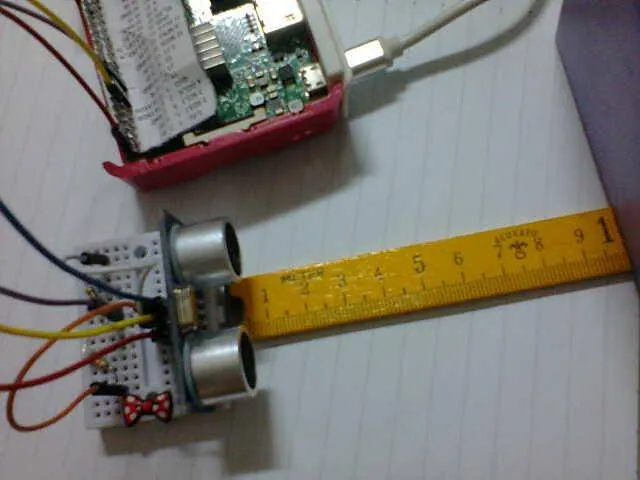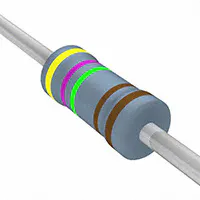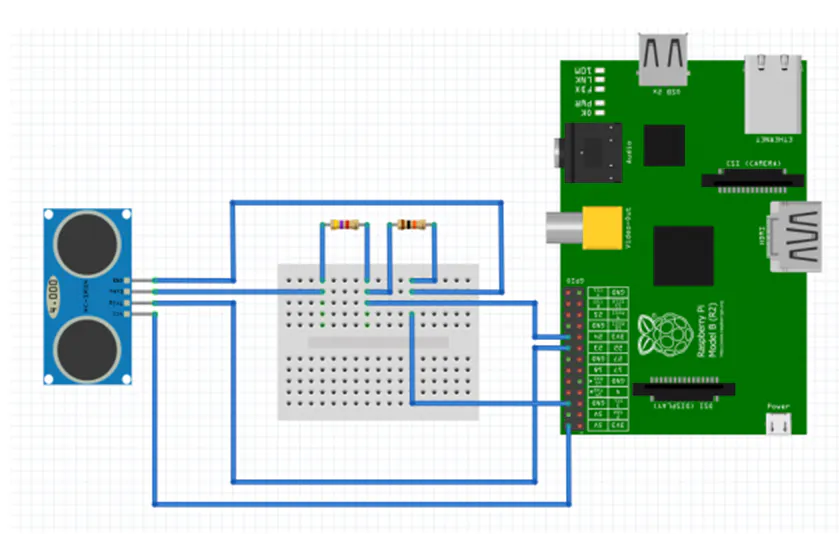Published

# Distance Measurement Using Python & Tk GUI Tools

This project uses an ultrasonic sensor to indicate the distance of any object from it.

BeginnerFull instructions provided1 hour2,853## Things used in this project

### Hardware componentsUltrasonic Sensor - HC-SR04 (Generic)
×1Raspberry Pi 3 Model B
×1Jumper wires (generic)
×1Resistor 10k ohm
×1Resistor 4.75k ohm
×1×1

PDF :

## Schematics

### Connecting To The Pi## Code

### The code 2 with start and stop button

Python
```from Tkinter import *
import RPi.GPIO as GPIO
import time
GPIO.setmode(GPIO.BOARD)
GPIO.setwarnings(False)
TRIG=11
ECHO=15
GPIO.setup(TRIG,GPIO.OUT)
GPIO.setup(ECHO,GPIO.IN,pull_up_down=GPIO.PUD_DOWN)
root=Tk()
def start():
global distance,pulse_end, p
TRIG=11
ECHO=15
GPIO.output(TRIG,False)
print("delay")
time.sleep(2)
GPIO.output(TRIG,True)
time.sleep(0.00001)
GPIO.output(TRIG,False)
while GPIO.input(ECHO)==0:
p=0
p=time.time()
while GPIO.input(ECHO)==1:
pulse_end=0
pulse_end=time.time()
d =pulse_end - p
distance=d*17150
#distance=round(distance,2)
print("Distance:",distance,"cm")
lable= Label (root,width=36,fg="yellow",bg="purple")
lable.config(font=("Courier",36))
lable.config(text=distance)
lable.pack()
button=Button(root,text='Stop',bg ='cyan',width=50,command=root.destroy)
button.pack()
button2=Button(root,text='Sart', bg ='gold',width=50,command=start)
button2.pack()
root.mainloop()
GPIO.cleanup()
```

### Measuring distance using Ultrasonic Sensor with Raspberry Pi code

Measuring distance using Ultrasonic Sensor with Raspberry Pi – Python Toolkit interface – HC-SR04

## Credits

### Aula Jazmati

17 projects • 27 followers